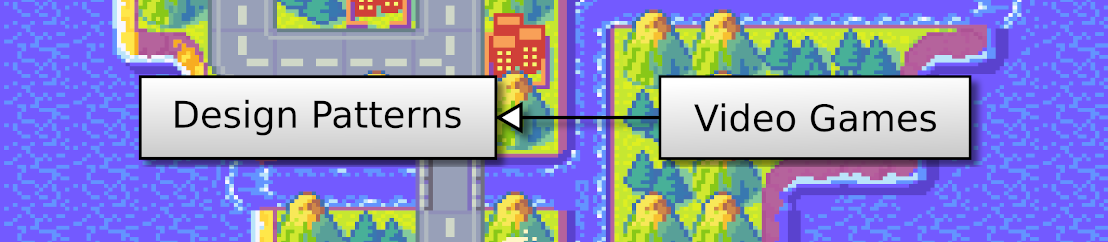## Discover Python and Patterns (9): Pygame

In the previous posts, I introduced enough of Python and patterns to start creating games with graphics using the Pygame library.

This post is part of the Discover Python and Patterns series

## Install Pygame

Pygame is a popular library for creating 2D games. Like the random library we used before, the import statement allows us to get access to all its features. However, the Anaconda environment we installed in the first post does not embed Pygame, and we have to install it.

To install a new python package, we need an Anaconda prompt. In Windows, type "Anaconda" in the start menu, and click "Anaconda Prompt". Then type the following command:

``pip install pygame``

You will get something similar to:

## First Pygame program

Here is a basic Pygame program that displays a window, and exits if we close it:

``````import pygame

pygame.init()
window = pygame.display.set_mode((640,480))

while True:
event = pygame.event.poll()
if event.type == pygame.QUIT:
break
pygame.display.update()

pygame.quit()``````

The first line imports the Pygame library. It gives access to all functions in the library. We can call all these functions preceding their name with `pygame.`. For example, line 3 calls the `init()` function that initializes the Pygame library. As for the `random` library, we could also use the following syntax:

``````from pygame import init
init()``````

With the `from ... import` syntax, we don't need to type `pygame.` before the name of a function. However, we must be sure that `init()` is the only function with that name. In most cases, I prefer the long syntax, because there is no doubt about which function I call.

Line 4 creates a window with a size of 640 per 480 pixels. This size does not include the window decorations. It is the size of the area where we draw our game.

Lines 6-10 is the main loop. Since we saw the Game Loop pattern in the previous post, this loop should look familiar. Lines 7-9 is the processing of inputs, and line 10 is the rendering. To handle inputs, Pygame stores all events (mouse moves, keyboard presses, etc.) in a queue. Then, we can read this queue using the function `pygame.event.poll()`. Note that each time we get an event, Pygame removes it from the queue (which is the normal behavior of a queue, but it is another story!).

The only event we process in this example is the one that asks for the end of the game. We read the `type` attribute of the event returned by `pygame.event.poll()`. These types are always values like `pygame.XXX`(or only XXX if you did a `from pygame import XXX`). I'll show several examples throughout this series.

Line 12 destroys the window.

## The for statement

With the previous program, we can only process one event per update, which can lead to latencies. We can also ask for all the currently available events using the function `pygame.event.get()`:

``````running = True
while running:

eventList = pygame.event.get()
for event in eventList:
if event.type == pygame.QUIT:
running = False

pygame.display.update()

pygame.quit()``````

In the program above, we store the list of events into the variable `eventList`. Note that `pygame.event.get()` removes all current events, so next time we'll get new ones.

To read all the elements of a list, we can use the `for` statement. The syntax is very simple:

``````for item in list:
...block...``````

`item` is the name of the variable that contains one element in `list` (a copy or a reference). The name of this variable is free, as long as it is not already used. For instance, if we run the following program:

``````list = [1,2,3]
for item in list:
print(item)``````

It displays:

``````1
2
3``````

For each value in the list, Python repeats the `for` block, where `item` has a different value. Note that `item` does not contain a value of the list. You can change the value of `item`, but it does not change the values in the list.

In the case of the Pygame event list, for each event in the list, if the event type is `pygame.QUIT`, we set the variable `running` to `False` to stop the main loop:

``````    for event in eventList:
if event.type == pygame.QUIT:
running = False``````

Note that we can't use the `break` statement to stop the main loop in the `for` loop. If we use the `break` statement in the `for` loop, it stops the `for` loop, but not the main loop that includes it. So, I used a variable as I did before in the "guess the number" game.

## Drawing

Pygame offers many functions for drawing, for instance, you can draw rectangles using the `pygame.draw.rect()` function:

``pygrame.draw.rect(surface, color, rect)``

The arguments are:

• `surface`: a Pygame object where we can draw. The window we created in the previous program is such a surface.
• `color`: a tuple with three integer values: amount of red, green, and blue. Values are between 0 (no color) and 255 (maximum). For instance `(255,0,0)` is the red color, and `(0,255,255)` is the purple color.
• `rect`: a tuple with four integer values: the top left x,y coordinates, the width, and the height of the rectangle. For instance `(10,20,200,100)` is a rectangle with x=10, y=20, width=200 and height=100.

We can use this function in our program to draw a blue rectangle in the center of the window. The function call is between the processing of inputs (the for events loop) and the update of the display:

``````import pygame

pygame.init()
window = pygame.display.set_mode((640,480))

running = True
while running:
for event in pygame.event.get():
if event.type == pygame.QUIT:
running = False
break

pygame.draw.rect(window,(0,0,255),(120,120,400,240))
pygame.display.update()

pygame.quit()``````

It leads to the following result:

Pygame documentation describes similar functions here. You should try some of these, for instance, draw a line or a circle, it will help you better understand the mechanics.

In the next post, I'll show you how to add user interaction with the keyboard.